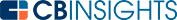# What is the Cap Rate Formula?A capitalization rate, or cap rate, is a value presented in percentage form. It’s a calculated formula used to determine the potential return on investment. Not only does it help investors determine profitability potential, but it also helps calculate time to break even.

The capitalization rate formula is commonly used for real estate investment purposes and is often a good gauge to determine which property may bring a bigger or faster return. It’s also useful to value an ongoing income stream, for business valuations, and to determine real estate market value.

## What is the cap rate formula used for?

The cap rate formula is used to help investors determine how much of a return they will make on a given investment, and how quickly they will break even. When investors buy real estate, for instance, they expect to make a profit in the form of ongoing income or resale value.

The same concept applies to any investment. The cap rate formula also helps to determine what a business might be valued at or to determine how long income can be derived from an investment asset.

## How to calculate cap rate

Calculating the cap rate of an asset or potential investment is as simple as dividing the net operating income (NOI) with the current market value.

For investment real estate property, you first calculate the property’s NOI by subtracting expenses from gross revenue. Divide that net income from the purchase price, then adjust that number by moving the decimal point two places to the right, or by multiplying by 100. This gives you the capitalization rate for the investment or asset in question as a percentage.

If you’d like to determine fair market value for an asset instead, you need the property’s net operating income and the current cap rate from similar assets. Then simply divide the NOI by the cap rate to determine the estimated value.

## How to calculate cap rate

Since capitalization rates are often used to evaluate real estate investments, let’s look at a real estate example to illustrate how the cap rate formula works.

Let’s say an investor intends to purchase real estate for \$100,000. The property has \$95,000 a year in expenses, thus the net operating income is \$5,000.

\$100,000 - \$95,000 = \$5,000

\$5,000 / \$100,000 = 0.05

Cap rate = 5% (0.05 x 100)

To determine the property value instead, you need to know the current going cap rate for similar properties and the existing property’s net operating income. Let’s say NOI is still \$5,000, but the cap rate on similar properties in the area is 6%.

\$5,000 / 0.06 = \$83,333.33

This implies that fair market value for the given property is a little over \$83,000.

When used for business valuations, the net operating income is replaced with the company’s profits, known as EBITDA (earnings before interest, taxes, depreciation, and amortization).

Generally the EBITDA used is the expected profits from the next year. Thus, if a company expects profits of \$100,000 the following year, and the cap rate for that industry is 20%, the cap rate formula is \$100,000 / 0.2, providing a valuation of \$500,000.

The cap rate formula is simply a calculation used for different types of investing. With real estate, the cap rate formula helps determine profitability and break even points on properties. Using this formula, investors can evaluate one property against another based solely on the rate of return. They also can use the formula to know how long it will take them to break even with the investment they’ve chosen.

For investors who are interested in acquiring or supporting closely held companies, the cap rate formula is a simple way to determine the valuation of the company they’re interested in.

You may also like# www.cbinsights.com